# How to Lock and Unlock Cells Based on Another Cell in Excel

Suppose we have a list of student names, and we want to lock this list if they fail the exam, and unlock them if they pass the exam, Fail or Pass will be saved in one cell for example A1, so if A1 is Fail, all cells with names will be locked, otherwise if A1 is Pass, these cells will be unlocked. To implement this function, we can via VBA code to lock and unlock cells based on another cell’s value.

Precondition:

See screenshots below: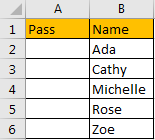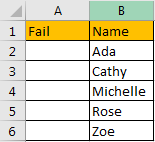Refer to different value in A1, we want to lock or unlock cell B2:B6.

## Method: Lock and Unlock Cells Based on Another Cell Value Via VBA Code

Step 1: On current visible worksheet, right click on sheet name tab to load Sheet management menu. Select View Code, Microsoft Visual Basic for Applications window pops up.

Or you can enter Microsoft Visual Basic for Applications window via Developer->Visual Basic. You can also press Alt + F11 keys simultaneously to open it.

Step 2: Enter below code in window:

```Private Sub Worksheet_Change(ByVal Target As Range)

If Range("A1") = "Pass" Then

Range("B2:B6").Locked = False

ElseIf Range("A1") = "Fail" Then

Range("B2:B6").Locked = True

End If

End Sub```

Step 3: Save the codes. And then quit Microsoft Visual Basic for Applications.

Step 4: Enter ‘Fail’ in A1, then select B2:B6, right click to load menu, select Format Cell. In Format Cells, click Protection tab. Verify that these cells are locked.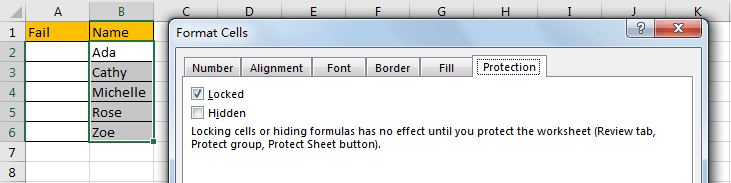Step 5: In ribbon, click Review tab, under Protect group click Protect Sheet.x
How to Create Dynamic Interactive Charts in Excel

Step 6: In Protect Sheet, enter password, click OK. Then confirm password.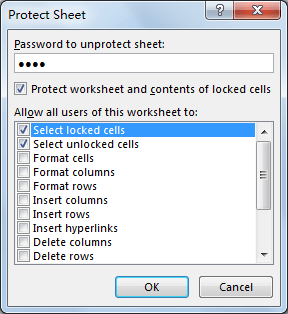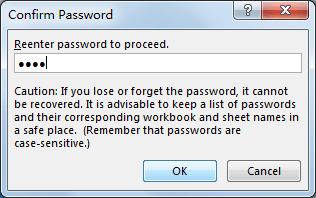Step 7: Now after above steps, worksheet is protected. Try to edit cell B2:B6.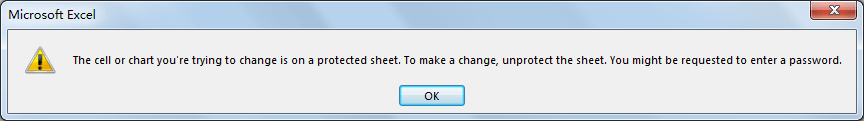Verify that warning message pops up.

Step 8: Go back to step#4, then enter ‘Pass’ in A1 this time, and protect worksheet again, verify that B2:B6 can be updated. (Please notice that ‘Run error’ may pops up sometimes, you can click ‘End’ to ignore the message.)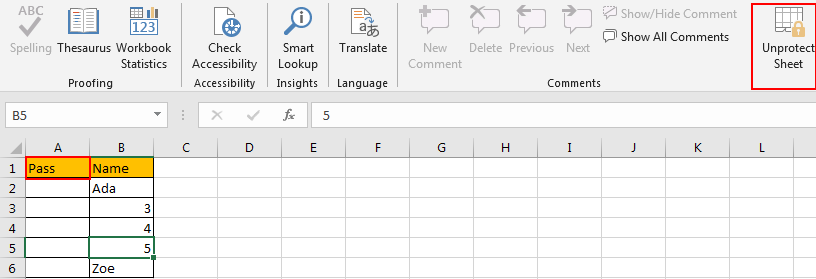Comment:

If value in A1 is not a text but a number for example 60, and if enter value <60, all cells are locked, you can enter below code:

```Private Sub Worksheet_Change(ByVal Target As Range)

If Range("A1").Value < 60 Then

ActiveSheet.Range("B2:B6").Locked = True

ElseIf Range("A1").Value >= 60 Then

ActiveSheet.Range("B2:B6").Locked = False

End If

End Sub```

Check the result, see screenshots below:

Enter 59 in A1: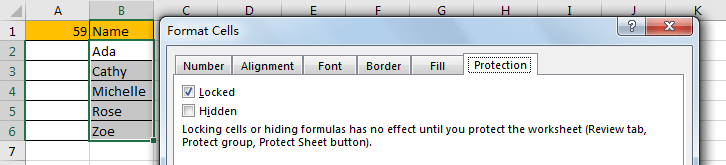Enter 60 in A1: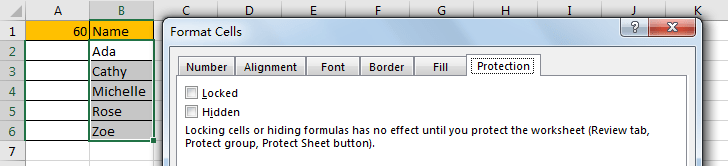Related Posts

VBA Macro For VLOOKUP From Another Sheet

In the previous post, you should know that how to fix or remove the #N/A error when using VLOOKUP formula to lookup value from another sheet. And this post will show you how to use VBA code to vlookup data ...

How To Insert Comments in Protected Worksheet in Excel

This post will show you how to allow comments in a protected worksheet in Excel. You can easily to insert comments into cells in a normal worksheet in Excel, but if want to insert a comment in a worksheet that ...

How To Convert Text to Upper Cases(Using VBA) in Excel

This post will show you how to switch from lower case to upper case in Excel. and I am going to show you two different ways of converting text to upper cases using formula or VBA macro in Excel 2013,Excel ...

How To Hide Every Other Row in Excel (Using VBA)

This post will show you how to hide alternate rows or columns in Excel or how to hide every third, fourth, fifth row or column in Excel. If you want to hide every other row in your current worksheet, how ...

How to Disable the Save As Prompt in Excel

This post will show you how to use a VBA Macro to save an Excel file and overwrite any existing file without a prompt so that you are going to get the little window that says file already exists do ...

How to Count Cells that Contain even or odd numbers in Excel

This post will guide you how to count the number of cells that contain odd or even numbers within a range of cells using a formula in Excel 2013/2016.How do I count cells that contain odd numbers through the use ...

How to Count Cells that Contain negative Numbers in Excel

This post will guide you how to count the number of cells that contain negative numbers within a range of cells using a formula in Excel 2013/2016.You can count the number of negative numbers in your data using easy functions ...

How to Count Cells Are Not Blank or Empty in Excel

This post will guide you how to count cells that are not blank or empty in a given range cells using a formula in Excel 2013/2016.How do I count the number of cells that are not blank in a particular ...

How to Count Cells Less Than a Specific Value in Excel

This post will guide you how to count the number of cells less than a particular numeric value in a given range cells using a formula in Excel 2013/2016. How do I count cells that are less than a specific ...

How to Count Cells Greater Than a Specific Value in Excel

This post will guide you how to count the number of cells greater than a particular numeric value in a given range cells using a formula in Excel 2013/2016. How do I count cells that are greater than a specific ...

Sidebar